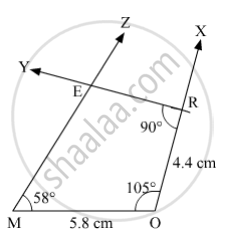SSC (English Medium) Class 8Maharashtra State Board
Share

# Construct the Following Quadrilateral of Given Measures. in ☐ More, L(Mo) = 5.8 Cm, L(Or) = 4.4 Cm, M∠M = 58°, M∠O = 105°, M∠R = 90° . - SSC (English Medium) Class 8 - Mathematics

#### Question

Construct the following quadrilateral of given measures.

In ☐ MORE, l(MO) = 5.8 cm, l(OR) = 4.4 cm, m∠M = 58°, m∠O = 105°, m∠R = 90° .

#### Solution

Steps of Construction:

Step 1:
Draw MO = 5.8 cm.

Step 2: Draw ∠MOX = 105° .

Step 3: With O as centre and radius 4.4 cm, draw an arc cutting ray OX at R.

Step 4: Draw ∠ORY = 90° .

Step 5: Draw ∠OMZ = 58° .  such that ray MZ and ray RY intersect each other at E.Here, MORE is the required quadrilateral.

Is there an error in this question or solution?

#### APPEARS IN

Balbharati Solution for Balbharati Class 8 Mathematics (2019 to Current)
Chapter 8: Quadrilateral : Constructions and Types
Practice Set 8.1 | Q: 1 | Page no. 43
Solution Construct the Following Quadrilateral of Given Measures. in ☐ More, L(Mo) = 5.8 Cm, L(Or) = 4.4 Cm, M∠M = 58°, M∠O = 105°, M∠R = 90° . Concept: Construction of a Quadrilateral.
S